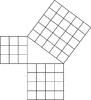# Geometric Pythagorean Theorem ProofIllustration that can be used to prove the Pythagorean Theorem, the sum of the squares of the legs is equal to the square of the hypotenuse. The geometrical illustration depicts a 3,4,5 right triangle with the square units drawn to prove that the sum of the squares of the legs (9 + 16) equals the square of the hypotenuse.

### Galleries

Geometric Theorems and Proofs

### Source

Florida Center for Instructional Technology Clipart ETC (Tampa: University of South Florida, 2007)

EPS (vector)

351.7 KiB

TIFF (full resolution)

2731×3000, 214.8 KiB

Large GIF

932×1024, 33.2 KiB

Medium GIF

582×640, 19.8 KiB

Small GIF

291×320, 8.6 KiB